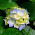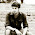Saturday, 12 November 2011

Class 10 - Light Reflection and Refraction (Short Q & A)

Light Reflection and Refraction
(Short Q & A)

Q1: In which type of lens linear magnification is always less then one?

Answer: Concave Lens always has linear magnification less than one, because it always gives diminished images.

Q2: John obtained a magnification of 1 with concave mirror of focal length 10cm. What is the object distance from mirror?

Answer: m = 1. Therefore u = v. Apply it in mirror formula, you will get v = 20 cm.

Q3: Which mirror has a wider field of view?

Answer: convex mirrors have wilder field view, that's why these are used as rear view-mirrors in vehicles.

Q4: Which is optically denser out of the two mediums M1 and M2 having n = 1.71 and 1.36
respectively.

Answer: M1 (it has higher value of refractive index).

Q5: Identify a mirror having focal length –15cm

Answer: concave mirror (see -ve sign of focal length).

Q6: Why does a ray change its path when it passes from one medium to another medium?

Answer: Because of refractive property of light.

Q7: When a ray of light entering from air is incident on the surface of a glass slab at an angle
of 90°, what will be the measure of angle of refraction?

Answer: It will be zero degree.

Q8: Due to which property of light, sharp shadows of opaque objects are obtained?

Answer: Since light travels in straight lines, any obstacle obstructing the path will cast its shadow.

Q9: Two medium with refractive index 1.31 and 1.50 are given. In which case
(i) Bending of light is more                               (ii) speed of light is more

Answer: (i) 1.50 and ii) 1.31

Q10
: Why does the coin placed at the bottom of a container appears to be raised when the
container is filled slowly with water?

Answer: Light ray emerging from water medium to air medium bends away and then reaches the eye.

Q11: Under what circumstances there won’t be any refraction of light when it enters from one
medium to another?

Answer: There are two possible cases:
(i) When light incidents at 90 degress (normal) it will not bend.
(ii) Only applicable when light passes from optical denser medium to rarer medium, if it is incident at an angle greater than critical angle, it will reflect (called internal reflection) but not refract.

Q12: Can a plane mirror ever form a real image?

Answer: Real image can only be formed when the reflected rays converge. As such for plane mirrors it is not possible.  However, if the reflected yas are converged (or appears converged), it can form real image. Consider a source of light is at infinity (say sun), and plane mirror is very small in size, its reflected image will show a circular bright spot (image of the sun) on the screen.

Q13: What feature of light corresponds (analogous) to loudness of sound?

Q14(CBSE): Identify types of mirror without touching.

Answer: Move mirror to and fro.
Plane Mirror: Mirror image is of true size.
Concave Mirror: Image is curved. Bringing the mirror closer, magnifies the image. Moving it away, image is inverted and reduced.
Convex Mirror: Mirror image is always diminished but erect. The viewpoint is wider.

Q15: What is the focal length of a plane mirror?

Q16: Will the reflected rays converge at point when a parallel beam of light is incident on a concave mirror?

Answer: Yes it will converge at the focal point of concave mirror.

Q17: Name any use of Concave lens.

Answer: Concave lens are mainly used in spectacles for the correction of shortsightedness.

Q19: Name the type of mirror which always forms virtual and diminished  image.

Q20: What is the angle of reflection if a ray falls normally on a plane mirror?

Q21: A ray of light travelling in air enters obliquely into water. Does the light ray bend towards the normal or away from the normal. Why?

Answer: When a ray of light travelling in air enters obliquely into water, it bends towards the normal. It happens because water is optically denser than air. Speed of light will also decrease.

Q22: What are the uses of convex mirror?

• rear-view mirror
• reflector of street light
• shopping malls and buildings have long paths have convex mirrors around the corner
Q23: List the uses of concave mirror?

• shaving mirror
• reflector in automobile lights and torches
• by dentists to see back side of tooth
• reflector of solar furnaces
Q24: How many images are formed for a point source kept in between two plane mirrors M1 and M2 at right angles to each other?

Q25: What shall be the direction of the incident ray which after reflection from a spherical mirror retraces its path?

Answer: In this case incident ray is directed towards the centre of curvature.

Q26: What are the characteristics of the image formed on a plane mirror?

Answer: Characteristics of Image formed on plane mirror are:
1. Image is upright and is of same size as the object.
2. It is virtual
3. Laterally inverted1.Please increase the number of questions on lenses! there is hardly any question on lenses.

2.One portion of a convex lens is covered as shown. Will the lens produces a complete image of the object? Describe in brief the characteristics of the image formed.

1.No , the image cannot be produced.

2.Image will be produced, but its intensity will decrease. You won't get a sharp and bright image.

3.There Will Be 2 Cases
1st Case: If optic center is Covered , ie more than half of the lens is covered , then image will not be formed.
Case 2 : If optic center is not covered , then the images will be formed clearly without any disorders

4.There Will Be 2 Cases
1st Case: If optic center is Covered , ie more than half of the lens is covered , then image will not be formed.
Case 2 : If optic center is not covered , then the images will be formed clearly without any disorders

5.the image will be formed if the object has been kept between the focus and twice the focal length i.e.between f and 2f. try it out

3.actually the complete image will be produced only the intensity of the image will be decreased.

4.1.5.6.